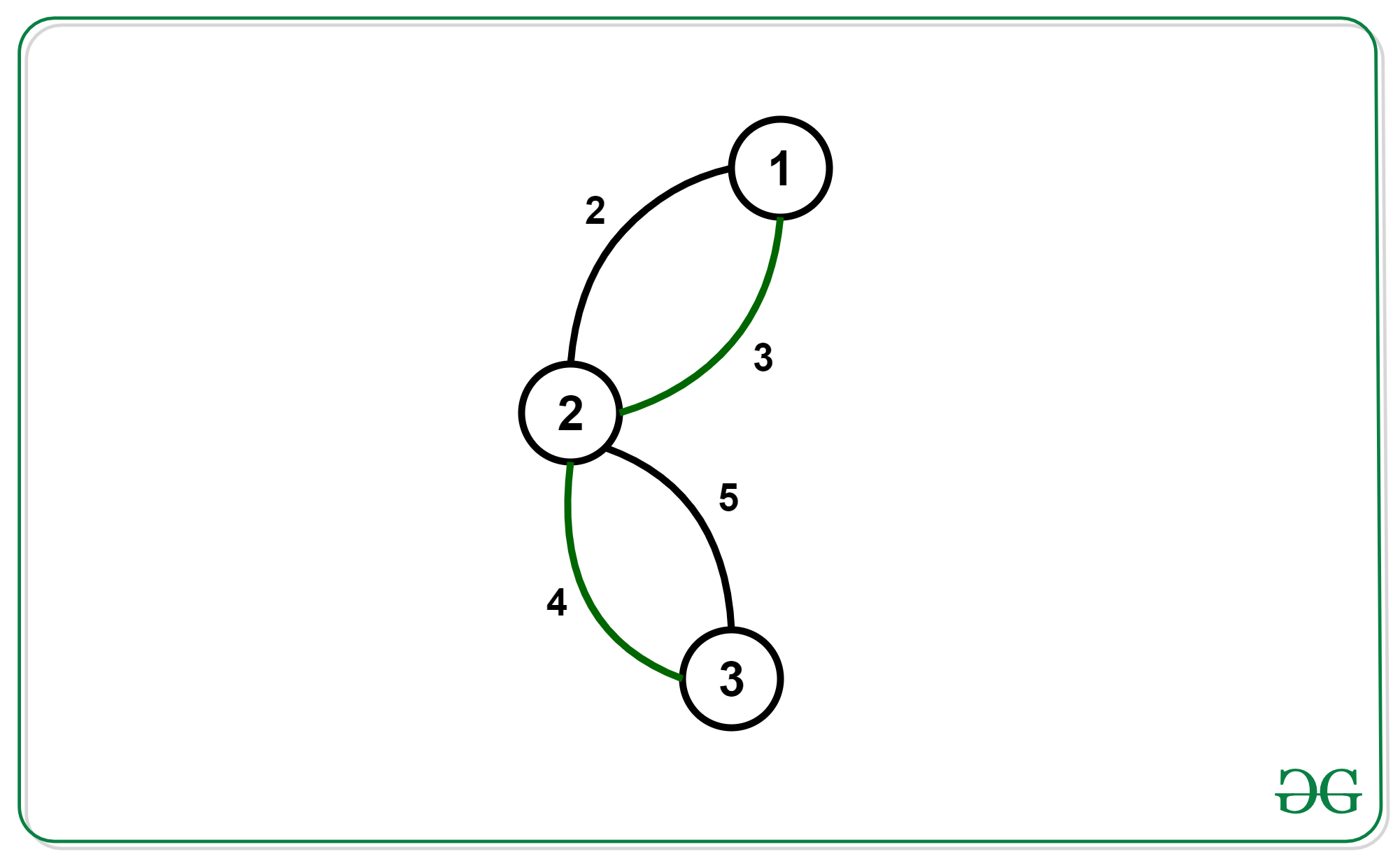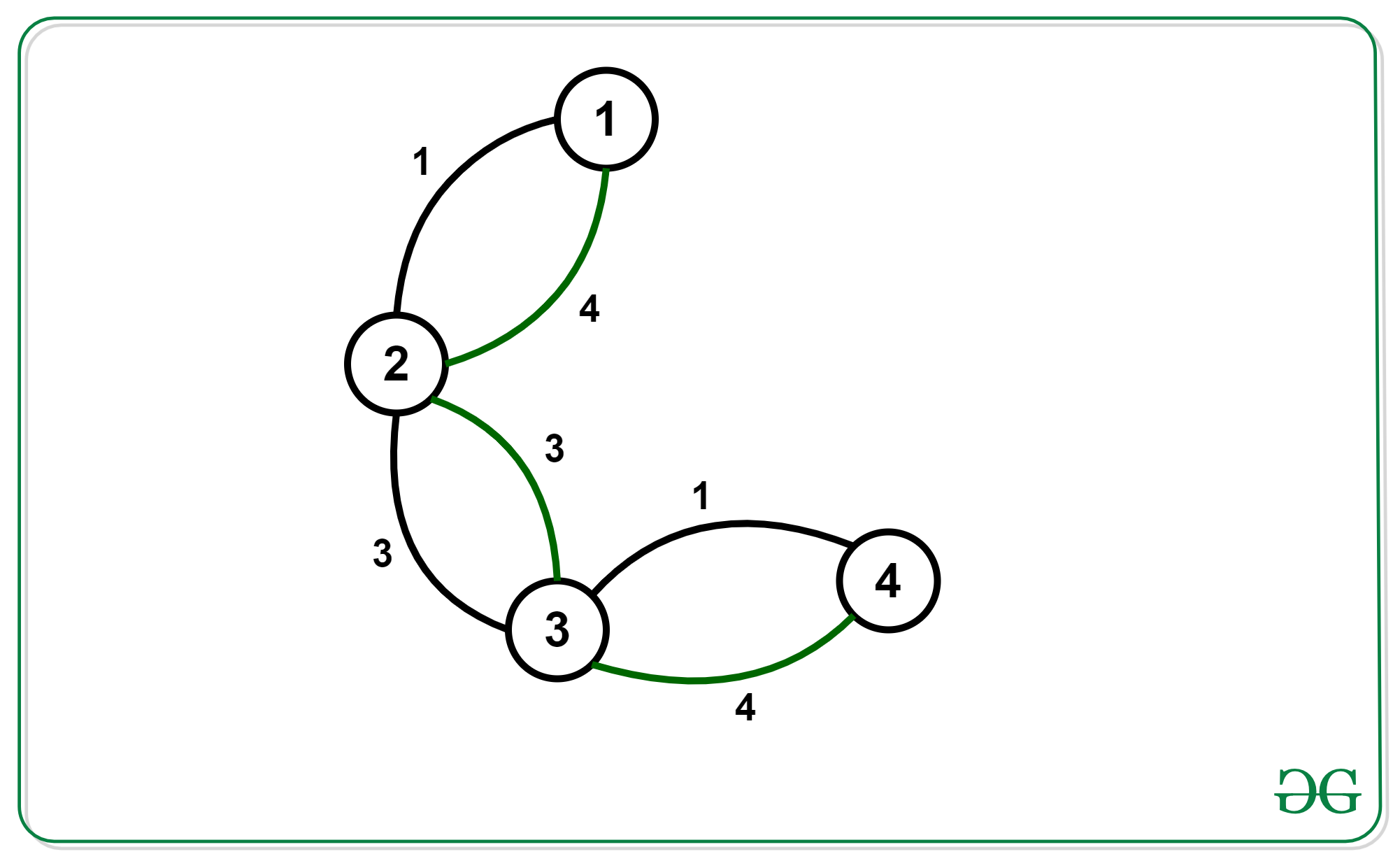# Find the minimum spanning tree with alternating colored edges

Given a graph with N nodes and M edges where each edge has a color (either black or green) and a cost associated with it. Find the minimum spanning tree of the graph such that every path in the tree is made up of alternating colored edges.

Examples:

Input: N = 3, M = 4Output: 6

Input: N = 4, M = 6Output: 4

## Recommended: Please try your approach on {IDE} first, before moving on to the solution.

Approach:

• The first observation we make here is every such kind of spanning tree will be a chain. To prove it, suppose we have a tree that is not a chain and every path in it is made up of alternating edges. Then we can deduce that at least 1 node has a degree of 3. Out of these 3 edges, at least 2 will have the same color. The path using these 2 edges will never follow the conditions and Hence, such kind of tree is always a chain.
• Now we can find a chain with minimum cost and alternating edges using bitmask-dp,
dp[mask(2^n)][Node(n)][col_of_last_edge(2)] where the mask is the bitmask of nodes we’ve added to the chain. Node is the last node we added to the chain.col_of_last_edge is the color of edge use to connect Node.
• To transition from 1 state to another state we visit the adjacency list of the last node and use those edges which have color != col_of_last_edge.

Below is the implementation of the above approach:

 `// C++ program for the ` `// above approach ` `#include ` `using` `namespace` `std; ` ` `  `int` `graph; ` ` `  `// Initializing dp of size = ` `// (2^18)*18*2. ` `long` `long` `dp[1 << 18]; ` ` `  `// Recursive Function to calculate ` `// Minimum Cost with alternate  ` `// colour edges ` `long` `long` `minCost(``int` `n, ``int` `m, ``int` `mask, ``int` `prev, ``int` `col) ` `{ ` `    ``// Base case ` `    ``if` `(mask == ((1 << n) - 1)) { ` `        ``return` `0; ` `    ``} ` `    ``// If already calculated ` `    ``if` `(dp[mask][prev][col == 1] != 0) { ` `        ``return` `dp[mask][prev][col == 1]; ` `    ``} ` ` `  `    ``long` `long` `ans = 1e9; ` ` `  `    ``for` `(``int` `i = 0; i < n; i++) { ` `        ``for` `(``int` `j = 0; j < 2; j++) { ` `            ``// Masking previous edges ` `            ``// as explained in above formula. ` `            ``if` `(graph[prev][i][j] && !(mask & (1 << i))  ` `                ``&& (j != col)) { ` `                ``long` `long` `z = graph[prev][i][j] +  ` `                              ``minCost(n,m,mask|(1<, ` `                      ``pair<``int``,``char``>>>& vp,``int` `m){ ` ` `  `  ``for` `(``int` `i = 0; i < m; i++) { ` `    ``int` `a = vp[i].first.first - 1; ` `    ``int` `b = vp[i].first.second - 1; ` `    ``int` `cost = vp[i].second.first; ` `    ``char` `color = vp[i].second.second; ` `    ``graph[a][b][color == ``'W'``] = cost; ` `    ``graph[b][a][color == ``'W'``] = cost; ` `  ``} ` `} ` ` `  `// Function to getCost ` `// for the Minimum Spanning ` `// Tree Formed ` `int` `getCost(``int` `n,``int` `m){ ` `     `  `    ``// Assigning maximum ` `    ``// possible value. ` `    ``long` `long` `ans = 1e9; ` ` `  `    ``for` `(``int` `i = 0; i < n; i++) { ` `        ``ans = min(ans, minCost(n, m, 1 << i, i, 2)); ` `    ``} ` ` `  `    ``if` `(ans != 1e9) { ` `        ``return` `ans; ` `    ``} ` `    ``else` `{ ` `        ``return` `-1; ` `    ``} ` `} ` ` `  `// Driver code ` `int` `main() ` `{ ` `    ``int` `n = 3, m = 4; ` `    ``vector, pair<``int``, ``char``> > > vp = { ` `        ``{ { 1, 2 }, { 2, ``'B'` `} }, ` `        ``{ { 1, 2 }, { 3, ``'W'` `} }, ` `        ``{ { 2, 3 }, { 4, ``'W'` `} }, ` `        ``{ { 2, 3 }, { 5, ``'B'` `} } ` `    ``}; ` ` `  `    ``makeGraph(vp,m); ` `    ``cout << getCost(n,m) << ``'\n'``; ` `     `  `    ``return` `0; ` `} `

Output:

```6
```

Time Complexity: O(2^N * (M + N))

My Personal Notes arrow_drop_upCompetitive Programmer | Python Developer

If you like GeeksforGeeks and would like to contribute, you can also write an article using contribute.geeksforgeeks.org or mail your article to contribute@geeksforgeeks.org. See your article appearing on the GeeksforGeeks main page and help other Geeks.

Please Improve this article if you find anything incorrect by clicking on the "Improve Article" button below.